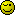# Searchresult from multiple parameters?!?!

I’m trying to make a search script where the user can set multiple parameters, but am not succesfull…

Here is what I have:

``````\$sql="SELECT c.ccountry
, c.cregion
, c.cclub
, c.created
, c.clubid
, c.creator
, c.seoname
, c.clubname
, cm.members FROM ".\$prefix."_club c
INNER JOIN
(SELECT COUNT(*) as members FROM ".\$prefix."_club_users WHERE clubid='c.clubid' ";
if((\$mem_low > 1) || (\$mem_high < 26)){
\$sql.="AND cm.members > \$mem_low AND cm.members < \$mem_high";
}
\$sql.=") cm ";
if(\$ccountry){
\$sql.="WHERE c.ccountry=\$ccountry ";
}
if((\$cstate) && (\$cstate != ''._CHOOSE.' '._STATE_REGION.'')){
\$sql.="AND c.cregion=\$cstate ";
}
if(\$ccourse){
\$sql.="AND c.cclub=\$ccourse ";
}
\$sql.="ORDER BY c.created DESC";
\$result = mysql_query(\$sql) or die("Error: (" . mysql_errno() . ") " . mysql_error());
\$r_count = 0;
while(\$row = mysql_fetch_array(\$result)){
``````

I get this error:
Error: (1054) Unknown column ‘cm.members’ in ‘where clause’

Can’t figure out how to get passed this…

Please helpI recommend first writing the query by hand. Get it working.

Then, you can use php to dynamically create some text that is the same as the working sql query. Now when you echo \$sql you can compare it to the working query and see the difference.

This will make it much easier on you.

But the error is in the subquery, there is no table or alias named cm. The result of the subquery is given the alias of cm.

Hmmm. Not quite sure what youi mean?

I recommend first writing the query by hand. Get it working.

Well… It works except the subquery part… I have also tryid this but with no luck…

``````\$sql.="AND members > \$mem_low AND members < \$mem_high";
``````

Hmmm Please helpHow about you show us the query? You’ve shown us php code, but this is an sql problem.

Oh yes… This should have been posted in the sql forum… Sorry.

Well, have been playing around a bit and figured out if I do this:

``````\$sql="SELECT c.ccountry
, c.cregion
, c.cclub
, c.created
, c.clubid
, c.creator
, c.seoname
, c.clubname
, cm.count FROM ".\$prefix."_club c
INNER JOIN
(SELECT COUNT(*) count FROM ".\$prefix."_club_users WHERE clubid='c.clubid') cm ";
if(\$ccountry){
\$sql.="WHERE c.ccountry=\$ccountry ";
}
if((\$cstate) && (\$cstate != ''._CHOOSE.' '._STATE_REGION.'')){
\$sql.="AND c.cregion=\$cstate ";
}
if(\$ccourse){
\$sql.="AND c.cclub=\$ccourse ";
}
if((\$mem_low > 1) || (\$mem_high < 26)){
if(\$ccountry){
\$sql.="AND cm.count > \$mem_low AND cm.count < \$mem_high ";
} else {
\$sql.="WHERE cm.count > \$mem_low AND cm.count < \$mem_high ";
}
}
\$sql.="ORDER BY c.created DESC";
``````

My problem is the “c.clubid” in the sql query inside… If I change this to a specific number it works fine… Why don’t I get this???